Real time trapezoidal shaping algorithm at high count rates
HUANG Yuyan, GONG Hui, LI Jianmin
Key Laboratory of Particle and Radiation Imaging of Ministry of Education, Department of Engineering Physics, Tsinghua University, Beijing 100084, China
Abstract: A trapezoidal shaping algorithm was developed for common exponential nuclear signals and the pile-up effect at high count rate was studied. Deconvolution was used for time-of-arrival estimates to effectively improve the ability to recognize pile-up pulses and reduce the pile-up effect on the energy resolution. A complete pulse height analyzer was built in LabVIEW to realize real time trapezoidal shaping, pile-up recognition and spectrum construction in the field-programmable gate array (FPGA). Measurements with an X-ray diffractometer with a silicon drift detector (SDD) give a 2.46% energy resolution at a count rate of 1.8×105 cps (counts per second).
Key words: real time trapezoidal shaping     high count rate     pile-up effect     X-ray diffractometer

X射线衍射仪是一种常用的晶体结构分析仪器。本文实验中的射线源为铜的特征X射线，主要存在Kα和Kβ两种特征，对应能量分别为8.05和8.9 keV，由于后续晶体分析实验中只用到Kα峰，故需通过单道上下阈值的方式将Kβ峰去除，防止它对分析结果产生干扰。本文实验系统结构如图 1所示。图 1 X射线衍射实验系统结构

1 梯形成形算法在FPGA中的实现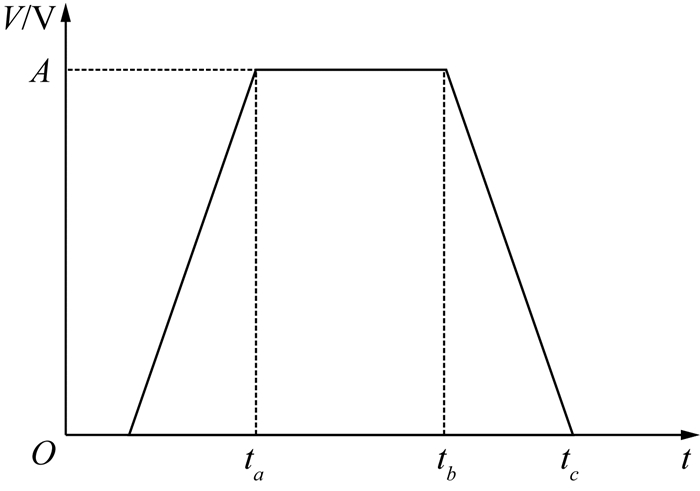图 2 理想梯形脉冲

 ${V_i}\left( t \right) = A{{\rm{e}}^{-t/\tau }}u\left( t \right).$ (1)

 $H\left( z \right) = \frac{{{z^{-1}}\left( {1-d{z^{-1}}} \right)\left( {1 - {z^{ - {n_a}}}} \right)\left( {1 - {z^{ - {n_b}}}} \right)}}{{{n_a}{{\left( {1 - {z^{ - 1}}} \right)}^2}}}.$ (2)
 $d = {{\rm{e}}^{-{T_{\rm{s}}}/\tau }},$ (3)
 ${n_a} = {t_a}/{T_{\rm{s}}}, {\rm{ }}{n_b} = {t_b}/{T_{\rm{s}}}, {\rm{ }}{n_c} = {t_c}/{T_{\rm{s}}}.$ (4)

H(z)=H1(z)H2(z)H3(z)H4(z).

 $\left\{ {\begin{array}{*{20}{c}} {{H_1}\left( z \right) = 1-{z^{-{n_b}}}, \;\;\;\;\;}\\ {{H_2}\left( z \right) = \frac{{1-d{z^{ - 1}}}}{{{n_a}\left( {1 - {z^{ - 1}}} \right)}}, }\\ {{H_3}\left( z \right) = \frac{{{z^{ - 1}}}}{{1 - {z^{ - 1}}}}, \;\;\;\;\;\;}\\ {{H_4}\left( z \right) = 1 - {z^{ - {n_a}}}.\;\;\;\;\;\;} \end{array}} \right.$ (6)

H1(z)与H4(z)相当于微分运算，H2(z)与H3(z)相当于积分运算。将微分H1放在第一级可防止溢出，微分H4放在最后一级有利于减小误差。H1H4的区别在于存储数据的长度，前者为nb，后者为na。考虑到FPGA中滤波器系数的误差，为了避免反馈误差积累，提高运算精度，在中间运算时将系数放大了217倍，因而后端数据占用更长位宽，故将H1放在第一级以减少所需的存储空间。H3子系统在运行过程中一直在累加数据，会产生溢出，故需在无信号到来的时间段对H3的输出以及H4中存储的历史数据进行清零。

2 堆积识别算法

 ${V_i}\left( t \right) = {{\rm{e}}^{-t/\tau }}u(t).$ (7)

 ${V_i}\left( z \right) = \frac{1}{{1-d{z^{-1}}}}.$ (8)

 $H\left( z \right) = \frac{{X\left( z \right)}}{{{V_i}\left( z \right)}} = 1-d{z^{-1}}.$ (9)

 $y\left( n \right) = x\left( n \right)-dx(n-1).$ (10)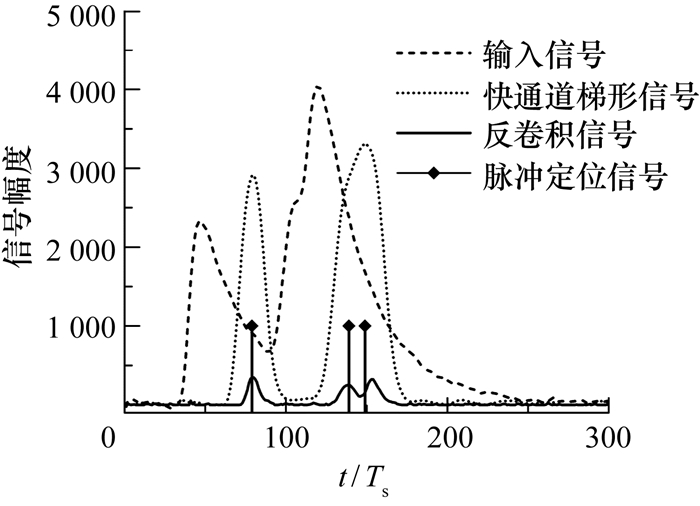图 3 堆积识别算法仿真波形

3 实验与应用结果

3.1 能谱与分辨率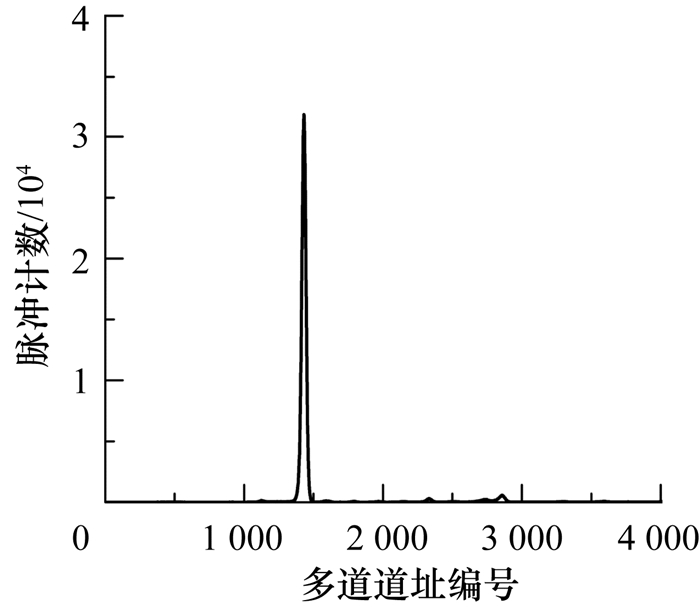图 4 8.05 keV铜Kα特征X射线能谱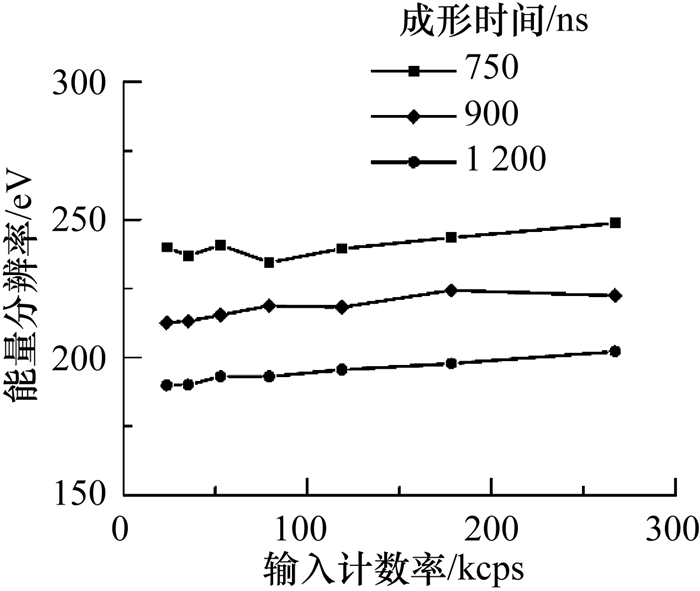图 5 不同计数率与成形时间下的能量分辨率

3.2 计数率校正

 $p\left( {x = n} \right) = \frac{{{\lambda ^n}{{\rm{e}}^{-\lambda }}}}{{n!}}.$ (11)

 $p(\Delta t \ge t) = {{\rm{e}}^{-\lambda }}.$ (12)

 ${p_{{\rm{record}}}} = {{\rm{e}}^{-2\lambda }},$ (13)
 $\lambda = {R_{{\rm{real}}}}{t_{{\rm{pile-up}}}}.$ (14)

 ${\rm{ }}{R_{{\rm{real}}}}.{{\rm{e}}^{-2{R_{{\rm{real}}}}{t_{{\rm{pile-up}}}}}} = {R_{{\rm{measure}}}}.$ (15)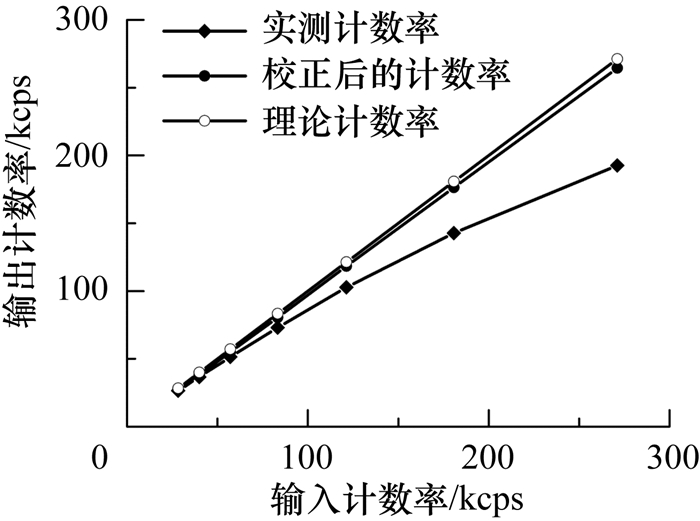图 6 校正前后脉冲输入计数率与输出计数率的关系

4 结论

  Radeka V. Trapezoidal filtering of signals from large germanium detectors at high rates[J]. IEEE Transactions on Nuclear Science, 1972, 99(3): 525–539.  肖无云, 魏义祥, 艾宪芸. 数字化多道脉冲幅度分析中的梯形成形算法[J]. 清华大学学报:自然科学版, 2005, 45(6): 810–812. XIAO Wuyun, WEI Yixiang, AI Xianyun. Trapezoidal shaping algorithm for digital multi-channel pulse height analysis[J]. Journal of Tsinghua University: Science and Technology, 2005, 45(6): 810–812. (in Chinese)  Bolic M, Drndarevic V. Digital gamma-ray spectroscopy based on FPGA technology[J]. Nuclear Instruments & Methods in Physics Research, 2002, 482(3): 761–766.  Bolic M, Drndarevic V. Processing architecture for high count rate spectrometry with NaI(Tl) detector [C]//IEEE Instrumentation and Measurement Technology Conference Record. Victoria, BC, Canada: IEEE Press, 2008: 274-278.  Cano-Ott D, Tain J L, Gadea A, et al. Pulse pileup correction of large NaI(Tl) total absorption spectra using the true pulse shape[J]. Nuclear Instruments & Methods in Physics Research, 1999, 430(2): 488–497.  Wong W H, Li H. A scintillation detector signal processing technique with active pileup prevention for extending scintillation count rates[J]. IEEE Transactions on Nuclear Science, 1998, 45(3): 838–842. DOI:10.1109/23.682647  Haselman M D, Pasko J, Hauck S, et al. FPGA-based pulse pile-up correction with energy and timing recovery[J]. IEEE Transactions on Nuclear Science, 2012, 59(5): 1823–1830. DOI:10.1109/TNS.2012.2207403  Ehrenberg J E, Ewart T E, Morris R D. Signal-processing techniques for resolving individual pulses in a multipath signal[J]. Journal of the Acoustical Society of America, 1978, 63(6): 1861–1865. DOI:10.1121/1.381926  Bolic M, Drndarevic V, Gueaieb W. Pileup correction algorithms for very-high-count-rate gamma-ray spectrometry with NaI(Tl) detectors[J]. IEEE Transactions on Instrumentation & Measurement, 2010, 59(1): 122–130.  LIU Zhenzhou, CHEN Jinxiang. A Monte Carlo based technique for analysing gamma-ray spectra[J]. Measurement Science & Technology, 2008, 19(8): 085102.  Meng L J, Ramsden D. An inter-comparison of three spectral-deconvolution algorithms for gamma-ray spectroscopy[J]. IEEE Transactions on Nuclear Science, 2000, 47(4): 1329–1336. DOI:10.1109/23.872973  Imperiale C, Imperiale A. On nuclear spectrometry pulses digital shaping and processing[J]. Measurement, 2001, 30(1): 49–73. DOI:10.1016/S0263-2241(00)00057-9  陈新光, 李翔宇, 孙义和. 粒子探测器读出电路数字滤波器设计[J]. 电子产品世界, 2010, 17(10): 29–31. CHEN Xinguang, LI Xiangyu, SUN Yihe. Particle detector readout circuits digital filter design[J]. Electronic Engineering & Product World, 2010, 17(10): 29–31. DOI:10.3969/j.issn.1005-5517.2010.09.006 (in Chinese)